## The Dirac Equation

We can extend this concept to use the relativistic energy equation (for now with no EM field). The idea is to replacewith.This is again written in terms of a 2 component spinor.

This equation is clearly headed toward being second order in the time derivative. As with Maxwell's equation, which is first order when written in terms of the field tensor, we can try to write a first order equation in terms of a quantity derived from. Define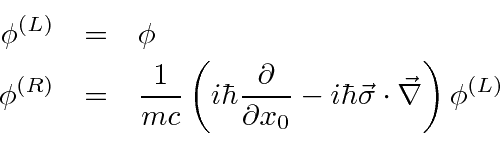Including the two components of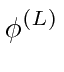and the two components of, we now have four components which satisfy the equations.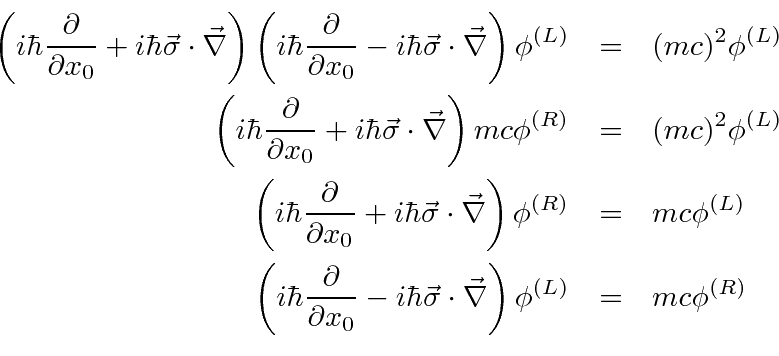These (last) two equations couple the 4 components together unless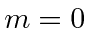. Both of the above equations are first order in the time derivative. We could continue with this set of coupled equations but it is more reasonable to write a single equation in terms of a 4 component wave function. This will also be a first order equation. First rewrite the two equations together, putting all the terms on one side.Now take the sum and the difference of the two equations.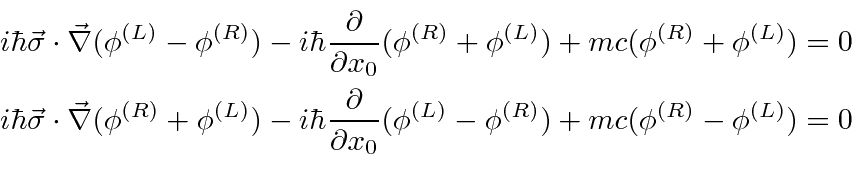Now rewriting in terms ofand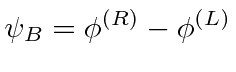and ordering it as a matrix equation, we get.Remember thatandare two component spinors so this is an equation in 4 components.

We can rewrite the matrix above as a dot product between 4-vectors. The matrix has a dot product in 3 dimensions and a time component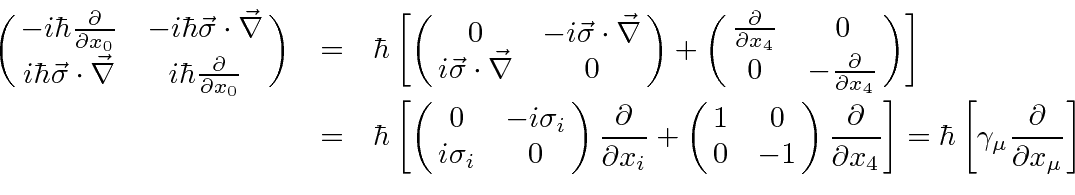The 4 by 4 matricesare given by.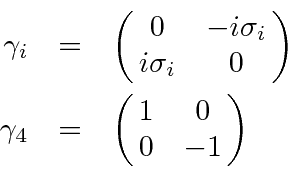With this definition, the relativistic equation can be simplified a great deal.The Dirac equation in the absence of EM fields isis a 4-component Dirac spinor and, like the spin states we are used to, represents a coordinate different from the spatial ones.

The gamma matrices are 4 by 4 matrices operating in this spinor space. Note that there are 4 matrices, one for each coordinate but that the row or column of the matrix does not correlate with the coordinate.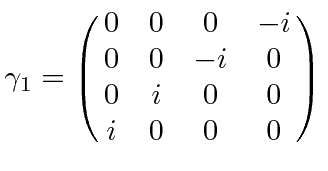Like the Pauli matrices, the gamma matrices form a vector, (this time a 4vector).

It is easy to see by inspection that thematrices are Hermitian and traceless. A little computation will verify that they anticommute as the Pauli matrices did.Sakurai shows that the anticommutation is all that is needed to determine the physics. That is, for any set of 4 by 4 matrices that satisfy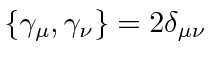,will give the same physical result
, although the representation ofmay be different. This is truly an amazing result.

There are a few other representations of the Dirac matrices that are used. We will try hard to stick with this one, the one originally proposed by Dirac.

It is interesting to note that the primary physics input was the choice of the Schrödinger-Pauli Hamiltonianthat gave us the correct interaction with the electron's spin. We have applied this same momentum operator relativistically, not much of a stretch. We have also written the equation in terms of four components, but there was no new physics in that since everything could be computed from two components, saysinceDirac's paper did not follow the same line of reasoning. Historically, the Schrödinger-Pauli Hamiltonian was derived from the Dirac equation. It was Dirac who produced the correct equation for electrons and went on to interpret it to gain new insight into physics.Dirac Biography

Jim Branson 2013-04-22